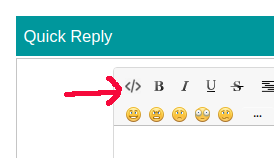# Millis???

Hi Guys,

I am very new to this and looking for a bit of assistance.

I want to check a value from a pot, and if it is in a particular range, I want it to delay (millis?) for 2seconds and check again. If the value is still in the same range, I want it to turn on a solenoid.

Sort of like a double check to make sure it is definitely in the range.

``````const int analogPin = A1;    // pin that the sensor is attached to
const int S1 = 12;       // Solenoid 1 on D12
const int S2 = 13;       // Solenoid 1 on D13
const int TooLow = 500;   // Sensor input is too low
const int TooHigh = 400;   // Sensor input is too high

void setup() {
// initialize the LED pin as an output:
pinMode(S1, OUTPUT);
pinMode(S2, OUTPUT);
// initialize serial communications:
Serial.begin(9600);
}

void loop() {
// read the value of the potentiometer:

// if the analog value is low enough, turn on Solenoid 1:
if (analogValue > TooLow) {

delay(1000);
if (analogValue > TooLow);
digitalWrite(S1, HIGH);
Serial.print ("Too Low ");

} else {
digitalWrite(S1, LOW);
}

// if the analog value is high enough, turn on Solenoid 2:
if (analogValue < TooHigh) {
digitalWrite(S2, HIGH);
Serial.print ("Too High ");
} else {
digitalWrite(S2, LOW);
}
// print the analog value:
Serial.println(analogValue);

delay(100);        // delay in between reads for stability

}
``````

The best way to check if a value stays the same throughout a period of time is to check when it is wrong - like this pseudo code

``````if (value is wrong) {
lastTimeValueWasWrong = millis();
}

if (millis() - lastTimeValueWasWrong >= timePeriod) {
// value has been correct throughout the period

// do something
}
``````

I'm not clear from your description as to exactly what test should be applied to tell if the value is wrong.

...R

PS ... When posting code please use the code button </>``````so your code
looks like this
``````

and is easy to copy to a text editor See How to use the forum

`if (analogValue > TooLow);`Oops

Right, 2 bugs in one line of codeFirst the old analogValue is checked, which does not reflect the current state, and then nothing is done even if the condition is true.

The Debounce example should shed some light onto the use of millis().

Thanks Robin2,

I am still a bit lost. I have added in what yo have said, however I am clearly doing something wrong.

exit status 1
'lastTimeValueWasWrong' was not declared in this scope

``````const int analogPin = A1;    // pin that the sensor is attached to
const int S1 = 12;       // Soleniod 1 on D12
const int S2 = 13;       // Soleniod 1 on D13
const int TooLow = 500;   // Sensor input is too low
const int TooHigh = 400;   // Sensor input is too high

void setup() {
// initialize the LED pin as an output:
pinMode(S1, OUTPUT);
pinMode(S2, OUTPUT);
// initialize serial communications:
Serial.begin(9600);
}

void loop() {
// read the value of the potentiometer:

// if the analog value is low enough, turn on Solenoid 1:
if (analogValue > TooLow) {
lastTimeValueWasWrong = millis();
}

if (millis() - lastTimeValueWasWrong >= timePeriod) {

digitalWrite(S1, LOW);  // value has been correct throughout the period
Serial.print ("Level ");

} else  {                 // do something
digitalWrite(S1, HIGH);
Serial.print ("Too Low ");
}

} else {
digitalWrite(S1, LOW);
}

// if the analog value is high enough, turn on Solenoid 2:
if (analogValue < TooHigh) {
digitalWrite(S2, HIGH);
Serial.print ("Too High ");
} else {
digitalWrite(S2, LOW);
}
// print the analog value:
Serial.println(analogValue);

delay(100);        // delay in between reads for stability

}
``````

go-r:
exit status 1
'lastTimeValueWasWrong' was not declared in this scope

You can't use a variable before you create it. Add:

``````unsigned long lastTimeValueWasWrong;
``````

to the top where you create your other variables.